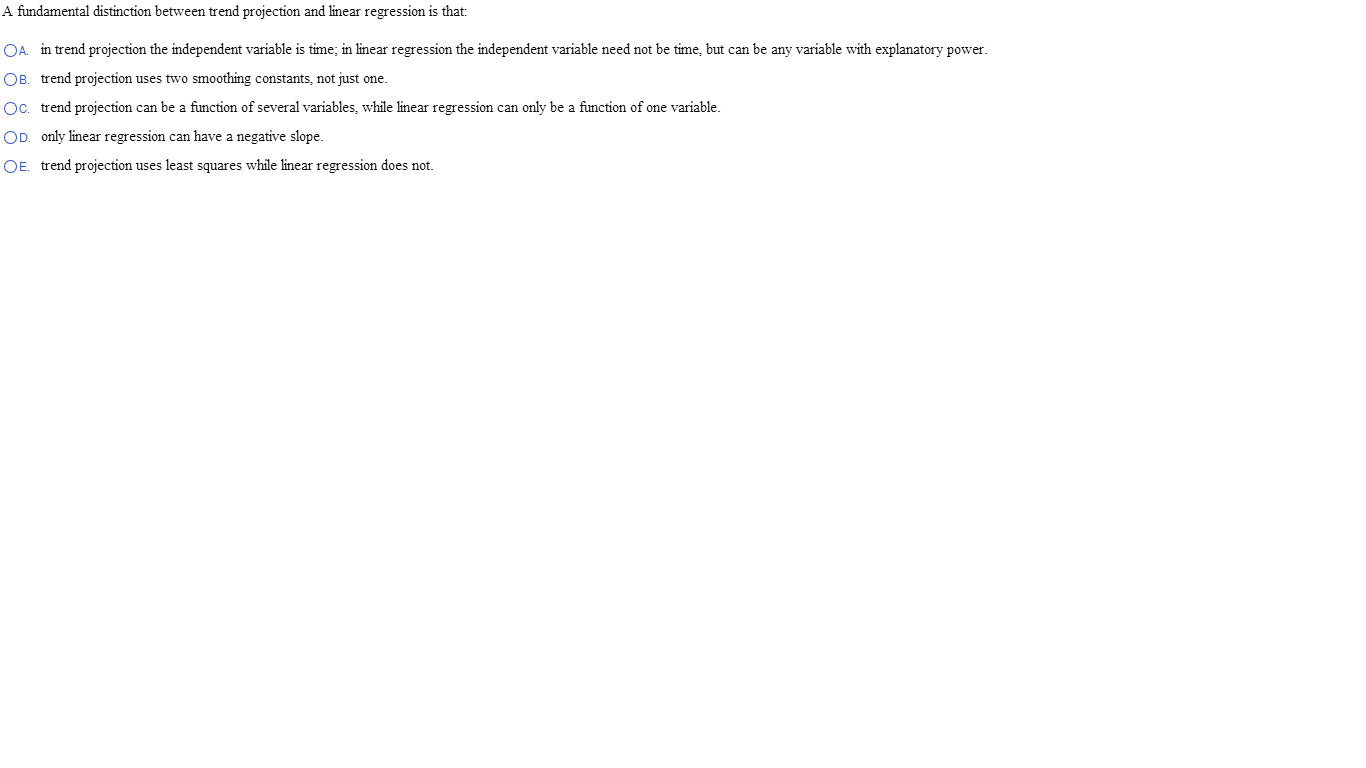# A fundamental distinction between trend projection and linear regression is that:A fundamental distinction between trend projection and linear regression is that: in trend projection the independent variable is time; in linear regression the independent variable need not be time, but can be any variable with explanatory power. trend projection uses two smoothing constants, not just one. trend projection can be a function of several variables, while linear regression can only be a function of one variable. only linear regression can have a negative slope. trend projection uses least squares while linear regression does not.

a) in trend projection the independent variable is time; in
linear regression the independent variable need not be time but can
be any expanatory variable